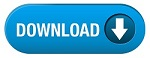NEW!

### Question Paper-2

Exam Name : Rajasthan PSC Main Exam (RAS/RTS Comb. Comp. Exam - 2016)

Total Marks : 200

Total Question : 65

Year: 2016

Subjects : Mathematics

:: Mathematics::

Identify the error/errors if any and wright the following Sentences :

Ques-1. Find the number of 5's in the following number chain such that sum of two digit before it is not greater than sum of two digits that follows it.

24593587652150503503

Ques-2. A particle can comleate circular round in one minute,but the move half round clockwise and one third round anticlockwise successively .Find the time in which particle will complete one round.

Ques-3. Find the smallest integer greater than 1 which is simultaneously a sqare and the cube of certain integers.

Ques-4. In a right angle tringle ABC,right angled at B, Prove that

(AB)3+(BC)3+(AC)3

Ques-5. What is the variance of first and natural number ?